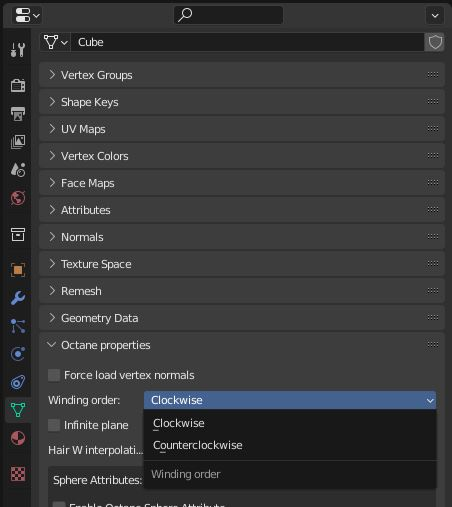# Winding Order

Determines how to control the Polygon normal input in the Mesh node. Set this parameter in a way that the polygon normals align with the vertex normal. This alleviates problems that occur when the polygon and vertex normals are pointing in the opposite directions, which happens with SpecularAmount of specular reflection, or the mirror-like reflection of light photons at the same angle. Used for transparent materials such as glass and water. materials and when applying displacement where the vertex or shading normals of displacement triangles are calculated during rendering using the polygon normals.Figure 1: Winding Order options